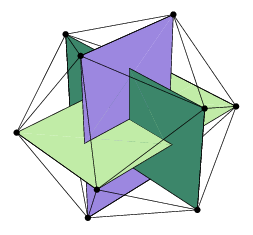# Icosahedron

IcosahedronClick on picture for large version.
TypePlatonic
Face polygontriangle
Faces20
Edges30
Vertices12
Faces per vertex5
Vertices per face3
Symmetry groupicosahedral (Ih)
Dual polyhedrondodecahedron
Propertiesregular, convex
Dihedral Angle138.1896852°

An icosahedron [ˌaıkəsə'hiːdrən] noun (plural: -drons, -dra [-drə]) is a polyhedron having 20 faces. The faces of a regular icosahedron are equilateral triangles. [Etymology: 16th Century: from Greek eikosaedron, from eikosi twenty + -edron -hedron], "icosa'hedral adjective

Missing image
Icosahedron_flat.png
image:icosahedron flat.png

In geometry, the regular icosahedron is one of the five Platonic solids. It is a convex regular polyhedron composed of twenty triangular faces, with five meeting at each of the twelve vertices. It has 30 edges. Its dual polyhedron is the dodecahedron.

The area A and the volume V of a regular icosahedron of edge length a are:

[itex]A=5\sqrt3a^2[itex]
[itex]V=\begin{matrix}{5\over12}\end{matrix}(3+\sqrt5)a^3[itex]Canonical coordinates for the vertices of an icosahedron centered at the origin are {(0,±1,±Φ), (±1,±Φ,0), (±Φ,0,±1)}, where Φ = (1+√5)/2 is the golden mean — note these form three mutually orthogonal golden rectangles. The 12 edges of an octahedron can be partitioned in the golden mean so that the resulting vertices define a regular icosahedron; the five octahedra defining any given icosahedron form a regular polyhedral compound.

There are distortions of the icosahedron that, while no longer regular, are nevertheless vertex-uniform. These are invariant under the same rotations as the tetrahedron, and are somewhat analogous to the snub cube and snub dodecahedron, including some forms which are chiral and some with Th-symmetry, i.e. have different planes of symmetry than the tetrahedron. The icosahedron has a large number of stellations, including one of the Kepler-Poinsot solids and some of the regular compounds, which could be discussed here.

Many viruses, e.g. herpes virus, have the shape of an icosahedron. Viral structures are built of repeated identical protein subunits and the icosahedron is the easiest shape to assemble using these subunits. A regular polyhedron is used because it can be built from a single basic unit protein used over and over again; this saves space in the viral genome.

In several roleplaying games, such as D&D, the twenty-sided die (for short, d20) plays a vital role in determining success or failure of an action.

If each edge of an icosahedron is replaced by a one ohm resistor, the resistance between opposite vertices is 0.5 ohms, and that between adjacent vertices 11/30 ohms.

### Icosahedron vs dodecahedron

Despite appearances, when an icosahedron is inscribed in a sphere, it occupies less of the sphere's volume (60.54%) than a dodecahedron inscribed in the same sphere (66.49%).

• Art and Cultures
• Countries of the World (http://www.academickids.com/encyclopedia/index.php/Countries)
• Space and Astronomy